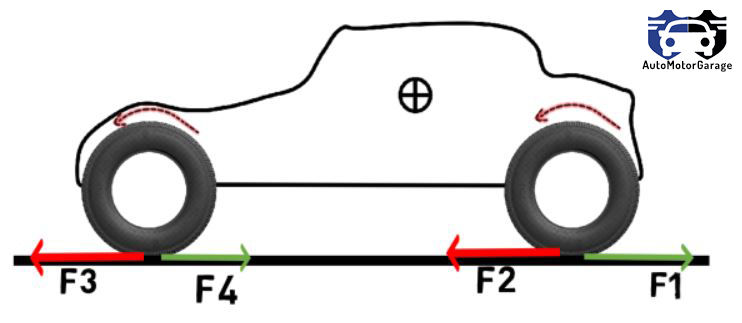top of page
Search

# What is Traction,it's direction & maximum value ?

Traction is the commonly used term and generally it is confused with friction.Friction is the big term as compare to traction.

Let's understand traction with example of rear wheel drive car.Above shown is the rear wheel drive car. As you press the accelerator pedal the rear wheel starts to rotate and pushes the ground with force F1 and then we get the reaction force F2 on tire which pushes the vehicle to move in forward direction. Now as the vehicle moves forward the front wheel pushes the ground with force F3 and gets the reaction force F4 which gives the torque in anticlockwise direction and rotates the wheel .So front tire moves with F3 and rotates by the torque produce by F4.Now consider the case their is no friction between the rear tire and ground.Will tire be able to push the ground with force F1. No ,off course. This is the similar case when you walk on the ground,While walking you push the ground and ground pushes you.If there was no friction between you and ground then you will slip. So, in case of car if their is no friction between tire and road then the rear tire will spin on its own axis and car will not go forward. Now if there is no friction between the front tire and ground then tire will not able to push the ground with force F3 and will not get the reaction force F4. So the front tire will not rotate and will skid on the ground.

So what is traction here, Traction is the force F2 on rear tire as the car is rear wheel drive and because of F2 the car is able to move forward.

Traction is the useful friction that acts between the drive wheels and ground. If you loose traction then you will loose the grip.

Drive wheels are the wheels having power.

Note :- Tractive force acts on the rear wheels if the car is rear wheel drive car ,it acts on front wheel if car is front wheel drive & acts on all wheel if car is 4 wheel drive car.

The maximum value of traction is given by:-

Ff (max) = μ * Wf (If car is front wheel drive)

Fr (max) = μ * Wr (If car is rear wheel drive)

F=Ff + Fr (If car is all wheel drive car)

Wf= L2*W/L - (F-fr*W)*h/L (To know from where these values came click here.) Wr= L1*W/L + (F-fr*W)*h/L

Where, Ff > Traction on front tire Fr > Traction on rear tire μ > Friction coefficient between road & tire Wf > Weight transfer on front tire Wr > Weight transfer on rear tire

Solving above equations we get:-

Ff(max)=μ*W*(L2+fr*h)/(L+μ*h) Fr(max)=μ*W*(L1-fr*h)/(L-μ*h)

## Conclusion

Traction is the useful friction that acts between the drive wheels and ground. If you loose traction then you will loose the grip.

Maximum value of traction is :-

Ff(max)=μ*W*(L2+fr*h)/(L+μ*h)

Fr(max)=μ*W*(L1-fr*h)/(L-μ*h)

Traction depends on:-

1) Weight transfer